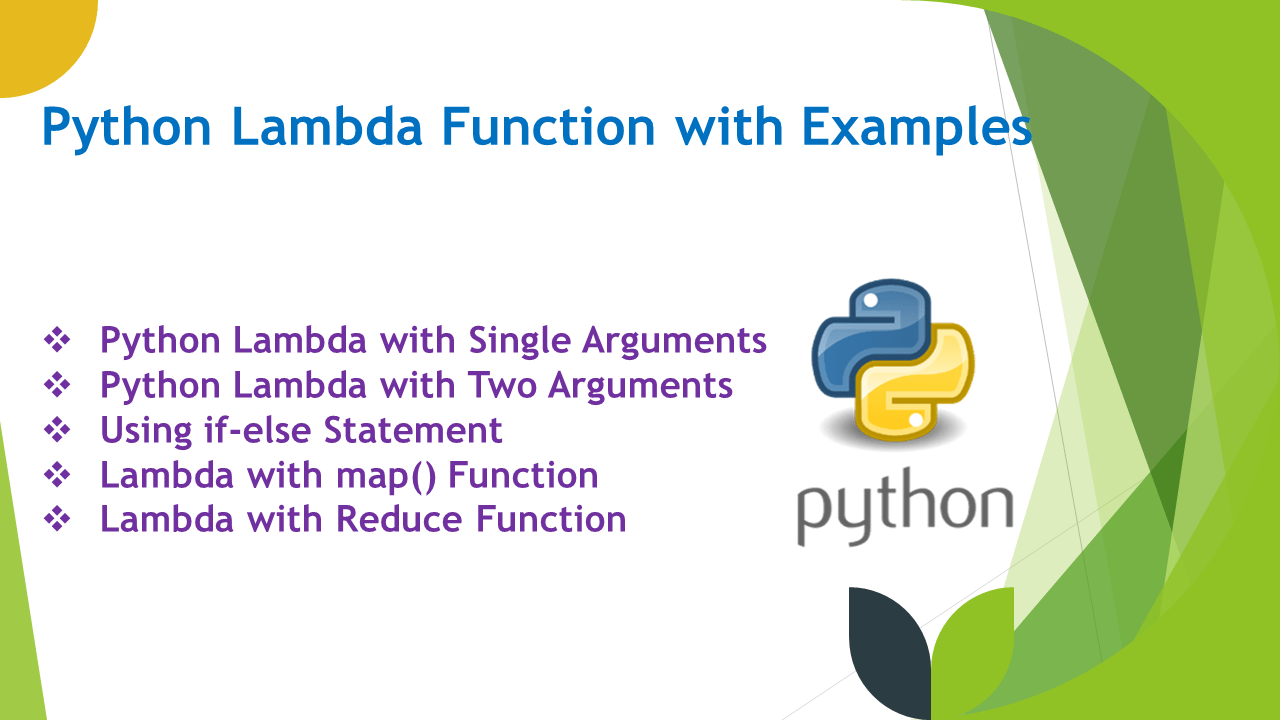# Python Lambda Function with Examples

A Python lambda operator or lambda function is a small anonymous function, an anonymous meaning function without a name. The Lambda functions are mainly used in combination with the functions filter(), map(), and reduce(). This function can take any number of arguments, but can only have one expression and they can be used wherever function objects are required in Python.

Key Points of Python Lambda Function:

• The `lambda` is a keyword to create a lambda function, similar to def which creates a python function.
• Lambda functions are anonymous functions, i.e functions without a name.
• This function can take multiple arguments and can have only one expression.
• The expression should be simple and short. For complex expressions, it is not recommended to use lambda
• You can also call a regular function from a lambda expression.
• This function is often used in conjunction with built-in functions such as filter(), map(), and reduce().
• Doesn’t use the return keyword.

## 1. Syntax of Lambda Function

Following is the syntax of the lambda function.

``````
# Syntax of lambda
lambda arguments: expression
``````

Here,

• `lambda` – is a python keyword that is used to create a lambda function.
• `arguments` – arguments to the lambda function.
• `expression` – expression to execute by using the arguments.

## 2. Python Lambda with Single Arguments

Python lambda function can take a single argument, executes the expression, and returns a result. In the below example, the lambda function is assigned to the variable `square`, you can call this function like any other function. The square function takes a single argument `x`, and returns the square of `x`.

``````
# Lambda example with single argument
square = lambda x: x * x
print(square(4))

# Output:
# 16
``````

## 3. Python Lambda with Two Arguments

Similarly, you can also create a lambda function with multiple arguments, let’s take another example with two arguments, add them and return the result.

``````
# Lambda example with two arguments
add = lambda x, y : x + y

#Output:
#30
``````

## 4. Using if-else Statement

You can also use an if-else statement in the lambda function. Here, is an example

``````
# Lambda function using if-else
min = lambda a, b : a if(a < b) else b
print(min(10, 20))

# Output:
# 10
``````

## 5. Lambda with map() Function

The map() function in Python is used to apply the transformation to an iterable object like a list, tuple, or set e.t.c, this applies the given transformation function to each item of an iterable (e.g., list, tuple, set). You can combine this with a lambda function. Here, is an example of using map() with lambda.

The `map()` is very useful when you want to apply a transformation to a large number of items without creating a new list, especially when you are working with big data.

``````
# Lambda with map()
numbers = [2, 4, 5, 6, 3]
squared_result = list(map(lambda x: x**2, numbers))
print(squared_result)

# Output:
# [4, 16, 25, 36, 9]
``````

In this example, the lambda function takes one argument `x` and returns its square. The `map()` function applies this lambda function to each item of the `numbers` list, and the result is a new iterator containing the squared values of each number. The `list()` function is used to convert the iterator to a list, so that we can print the results.

## 6. Lambda with Filter() Function

Let’s use the Python built-in function filter() with lambda to filter a list of values based on a given function.

In the below example, we are filtering out the even numbers from the list and converting the result into a list using the list() function. Here, the lambda function takes one argument `x` and returns `True` if `x` is even, `False` otherwise.

``````
# Lambda with filter()
numbers = [10, 11,12, 13, 14, 15, 16, 17, 18, 19, 20]
even_numbers = list(filter(lambda x: x % 2 == 0, numbers))
print(even_numbers)

# Output:
# [10,12, 14, 16, 18, 20]
``````

## 7. Lambda with Reduce Function

Finally, let’s use the lambda with reduce() function. The `reduce()` function applies a given function to the items of an iterable (e.g., list, tuple, set) in a cumulative way, and returns a single value. Here is an example of using lambda with reduce() function.

``````
# Lambda with reduce()
from functools import reduce
numbers = [1, 2, 3, 4, 5]
product = reduce(lambda x, y: x+y, numbers)
print(product)

# Output:
# 15
``````

This example adds all values in the list and returns a single value. In this example, the lambda function takes two arguments `x` and `y` and returns the sum of both. The `reduce()` function applies this lambda function cumulatively to the items of the `numbers` list, so first it will apply `1+2=3`, then `3+3=6`, then `6+4=10` and so on. The final result is a single value, the sum of all the numbers in the list.

## 8. Conclusion

In this article, you have learned what is lambda function and how to create an anonymous function and evaluate an expression. Also, we used the lambda function with python built-in function like map(), reduce(), and filter().

## Related Articles

### Naveen

I am a Data Engineer with 20+ years of experience in transforming data into actionable insights. Over the years, I have honed my expertise in designing, implementing, and maintaining data pipelines with frameworks like Apache Spark, PySpark, Pandas, R, Hive and Machine Learning. My journey in the field of data engineering has been a continuous learning, innovation, and a strong commitment to data integrity. I have started this SparkByExamples.com to share my experiences with the data as I come across. You can learn more about me at LinkedIn

### This Post Has One Comment

1.Anonymous

This is new for me, i think this so insteresting, thanks for content